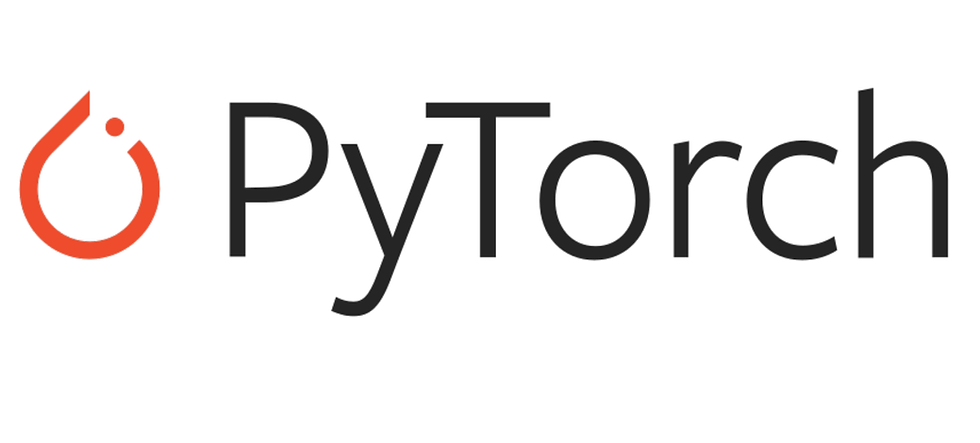top of page
Search

# Pytorch : Using Cross Entropy Loss With One Hot Encoded outputsCross Entropy Loss is one of the most popular loss functions for classification problems. Unlike most loss functions in deep learning, Cross Entropy Loss is one of few that requires two differently sized parameters to work correctly. Let's say you initialize the loss function like below:

```import torch
criterion = torch.nn.CrossEntropyLoss()```

In your training loop, to compute the loss, you will pass loss function ("criterion") two parameters to compute the loss and perform back prop. In my case, the two parameters passed are named outputs and targets where outputs is the output received from the Neural Network model and targets is the desired categorization:

```for X, targets in train_dataloader:
outputs = model(X)
loss = criterion(outputs, targets)
loss.backward()
optimizer.step()```

For Cross Entropy Loss to work correctly, the outputs from the network must be a one hot encoded vector of shape [N,C] where N is the batch size and C is the number of classes. Lets say we want to identify a dog, cat or no dog nor car in an image where index 0 is a no dog nor cat and index 1 is a dog, and index 2 is a cat. If we were to input four images (no dog nor cat image, dog image, cat image, cat image respectively) to our model, we want the model to produce an output of

```[[1,0,0],
[0,1,0],
[0,0,1],
[0,0,1]]```

which is a tensor of shape (4,3) since we have 4 images passed and 3 different classes to identify. The targets we want to pass to the loss function using the same no dog nor cat, dog, cat, cat images is

`[0,1,2,2]`

which is a tensor of shape (4) (batch size) and each element represents the index of the class we want to correctly categorize. To sum it all up in one sentence, in order for Cross Entropy Loss to work properly, the output from the neural network must be the one hot encoded vector of the target output. For more information about Cross Entropy Loss, please visit https://pytorch.org/docs/stable/generated/torch.nn.CrossEntropyLoss.html .